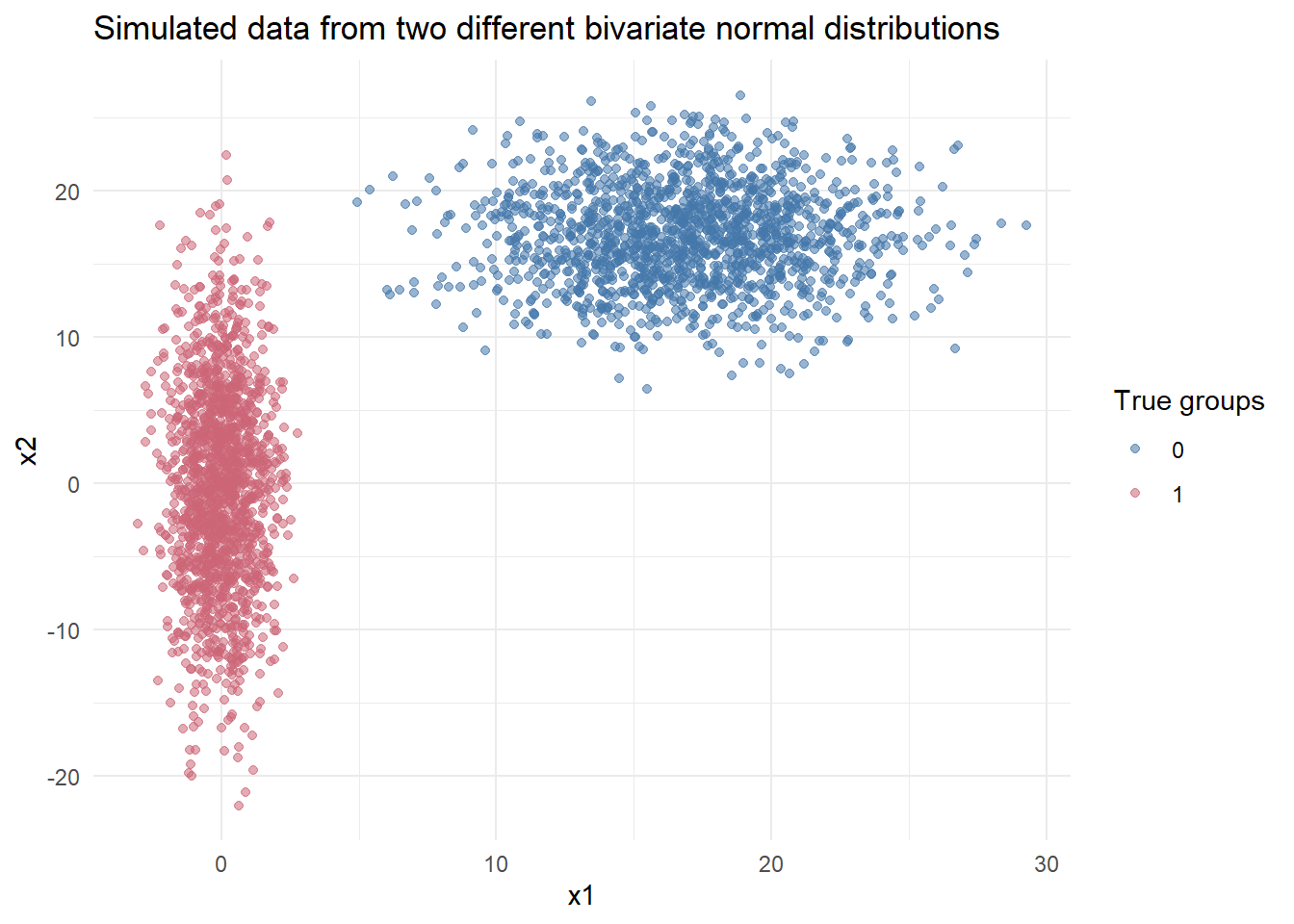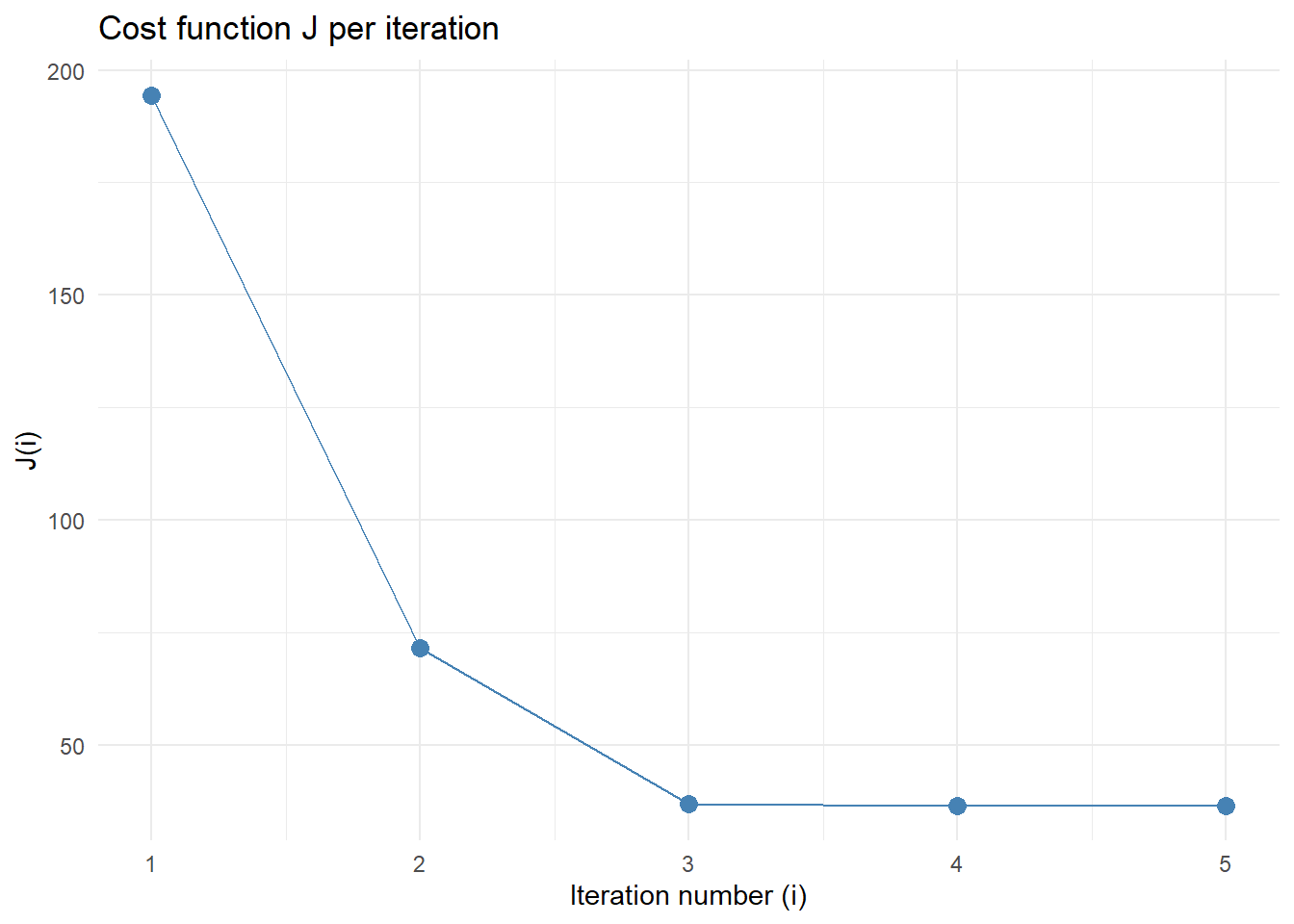# A brief post about k-means

###### PUBLISHED ON MAY 17, 2018 — ML, PYTHON

A few weeks ago I read the chapter 4 of book “Introduction to Applied Linear Algebra”, Boyd and Vandenberghe (2018), called “Clustering” and I found it so clear and simple. Chapter 4 introduce the clustering concept only based on vectors and distance –subjects treated on chapters 1 and 3 respectively–through the canonical example of clustering models: k-means.

In this post I want to make a little review of the chapter and implement k-means on python.

### Clustering

The idea of clustering is to partition a set of vectors into $$K$$ groups based on a distance measure. An intuitive way to think about it is thinking on a 2D-table where each observation (row) is a vector and we want to assign it to a cluster based on some similarity measure. So the goal is to add a new categorical variable (column) to the table with $$K$$ possible group values.

To draw the concept and to use an example of the chapter, imagine that we are in a hospital and we have a table with measures of a feature vector for each patient. A clustering could help to separate patients and get insights based on the groups. Maybe we could then assign labels or give a more elaborate description to the groups given the knowledge that we found.

To formalize the idea above:

• $$k$$: a parameter specifying the number of groups that we want to assign.
• $$c$$: the categorical variable with the group assignation, i.e. a vector with the size of the number of observations.
• $$G_i, i \in (1,\dots,k)$$: a set of indices that represent vectors assigned to group $$i$$.
• $$z_i, i \in (1,\dots,k)$$: a n-vector corresponding to group $$i$$ , this vector has the same length that vectors we want to assign a cluster.

The similarity within a group $$i$$ is given by the distance between vectors of a group $$i$$ and the group representative vector $$z_i$$. In which all members share the fact that the distance between this group representative ($$z_i$$) is the minimal with respect of another group representative’s vectors.

A simple measure of distance is the euclidean norm defined as:

$d(x, y) = ||x - y|| = \sqrt{\sum_{i=1}^{n}(x_i - y_i)^2}$

def euclidean_norm(x, y):
"""
x: a float numpy 1-d array
y: a float numpy 1-d array
---
Return the euclidean norm between x and y
"""
return np.sqrt(np.sum((x - y) ** 2))

The clustering objective function ($$J^{clust}$$) measures the quality of choice on cluster assignments. In the sense that a better cluster assignment of $$x_i$$ is when the square euclidean norm is lower.

$J^{clust} = (||x_1 - z_{c_1}||^2 + \dots + ||x_N - z_{c_N}||^2) / N$ Given the nature of the problem, find an optimal solution for $$J^{clust}$$ is really hard because depends on both group assignation ($$c$$) and the election of group representatives ($$z$$). Instead we can find a feasible solution for minimize $$J^{clust}$$, a suboptimal one (local optimum), solving a sequence of simpler optimization problems in an iterative approach.

### k-means algorithm

Now the way that the chapter shows how to assign these $$k$$ groups is through k-means algorithm. The best description for k-means is encapsulated in three steps:

1. Initialize with a set of fixed group of representatives $$z_i$$ for $$i = 1\dots k$$ (pick random observations of data as representatives for example)

2. Now we reduce the problem to just cluster assignations and also we can see this problem as $$N$$ subproblems (one for each observation). Just look what group of representatives has the minimal distance with vector $$x_i$$ and assign to vector $$c_i:=z^*$$. $||x_i - z_{c_i}|| = \underset{j=1, \dots, k}{\text{min}}\ ||x_i - z_j||$

3. The before step gives us a vector with the group assignation ($$c$$) of each observation ($$x_i$$). Remember that $$c$$ has the same length as number of observations that we have in the data. Now based on $$c$$, we come back to the problem of find a set of group representatives $$z$$. This step gives the name of k-means to the algorithm, because now we update the set of group representatives taking the mean of the vectors by cluster assigned in step 2. $z_j = \bigg(\frac{1}{|G_j|}\bigg)\underset{i\in G_j}{\sum{x_i}}$

I put the image of Ouroboros above as a graphical analogy, because k-means is self-contain and also iterative, steps 2 and 3 repeats. In each iteration a new assignation of clusters are given and then a redefinition of group representatives. But, opposite to the symbolic meaning of Ouroboros, the flow of time is not endless. The process of repetition ends when the algorithm converge to a solution.

When k-means reach a solution? The algorithm convergence occurs when there isn’t a variation in the group assignation with the previous iteration ($$c_t$$ is exactly the same that $$c_{t-1}$$).

The implementation of the three previous steps is very straightforward using numpy in python.

import numpy as np
def k_means(df, K, num_iter):
"""
df: a numpy 2D numpy array
K: number of group representatives
num_iter: number of iteration to repeat step 2 and 3
---
Return cluster assigned (c), group representatives (z) and cost function of
each iteration (J)
"""
nrow = df.shape
# initialize cluster representatives (STEP 1)
z = df[np.random.choice(nrow, K), ]
c = np.empty(nrow, dtype = 'int64')
J = []
iter_counter = 0
while True:
J_i = 0
# now solve the N subproblems of cluster assignation (STEP 2)
for j in range(nrow):
distance = np.array([euclidean_norm(df[j, ], z_i) for z_i in z])
c[j] = np.argmin(distance)
J_i += np.min(distance) ** 2
J.append(J_i / nrow)       # cost function evolution by iteration
# update group representatives, take the mean based on c (STEP 3)
check_z = np.array([np.mean(df[c == k, ], axis = 0) for k in range(K)])
if np.all(np.equal(check_z, z)):       # check convergence status
# reach convergence
break
if num_iter == iter_counter:
# stop process in iteration number -> iter_counter
break
z = check_z
iter_counter += 1
return c, z, J

A good idea to understand k-means is visually and step by step! So I wrote k_means with the argument num_iter that enables to stop the process in a certain iteration and get the results at that point.

We can play with the algorithm and show the evolution of centroid definition and cluster assignation, but before that we need some data. We generate two groups of random data $$D\in R^2$$ from two bivariate normal distributions with the following parameters:

$\mu_0 = (0, 0) \ \mu_1 = (17, 17) \\ \sigma_0 = \begin{bmatrix} 1 & 0 \\ 0 & 50 \\ \end{bmatrix} \ \sigma_1 = \begin{bmatrix} 15 & 0 \\ 0 & 12 \end{bmatrix}$

A simple data with 2 features helps us to visualize easily. Aditionally we know a priori that are two underlying groups, these are defined by the two different distributions and, if we take a look to the next plot, the two samples from these distributions are very well separated. Of course this is a very basic setting but the purpose is to ilustrate how k_means works.So k_means is blind to the colours and his goal is undercover the samples generated from the two underlying distributions based on data. Pictorically the process is as follows:For the cost side, $$J$$ decay rapidly and converge around a cost value of 36.

##  194.42629  71.55989  36.85485  36.60745  36.60636